# Enhanced Super-Resolution GAN Trained onDIV2K, Flickr2K and OST Data

Increase the resoution of an image

Released in 2018, this architecture uses the GAN framework to train a very deep network that both upsamples and sharpens an image. It is based on the SRGAN architecture but makes use of a number of improvements to model architecture and training, such as Residual-in-Residual Dense Blocks and Relativistic GAN training, to achieve better visual quality.

Number of layers: 1,093 | Parameter count: 16,697,987 | Trained size: 72 MB |

## Training Set Information

• The main dataset used for training, Diverse 2K (DIV2K), contains 800 2K resolution images. The Flickr2K and OutdoorSceneTraining (OST) datasets were used to enrich the training set with more diverse textures. The training data was augmented with random horizontal flips and 90-degree rotations.

## Performance

• This model achieves a peak signal-to-noise ratio of 27.03 and a perceptual index of 0.8153 on the Urban 100 dataset with a scale factor of 4.

## Examples

### Resource retrieval

Get the pre-trained net:

 In:=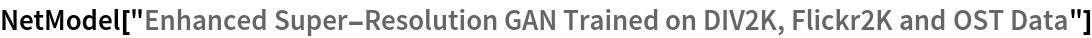Out=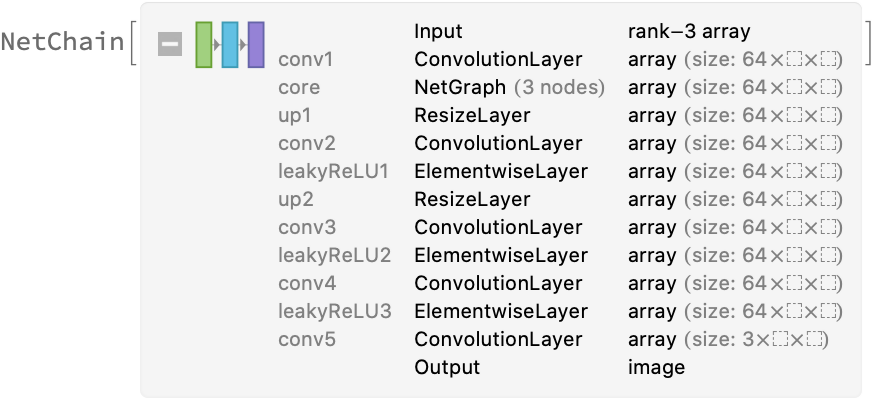### Evaluation function

ESRGAN has been trained to upscale images by a factor of 4. Create an evaluation function to handle any resize factor:

 In:=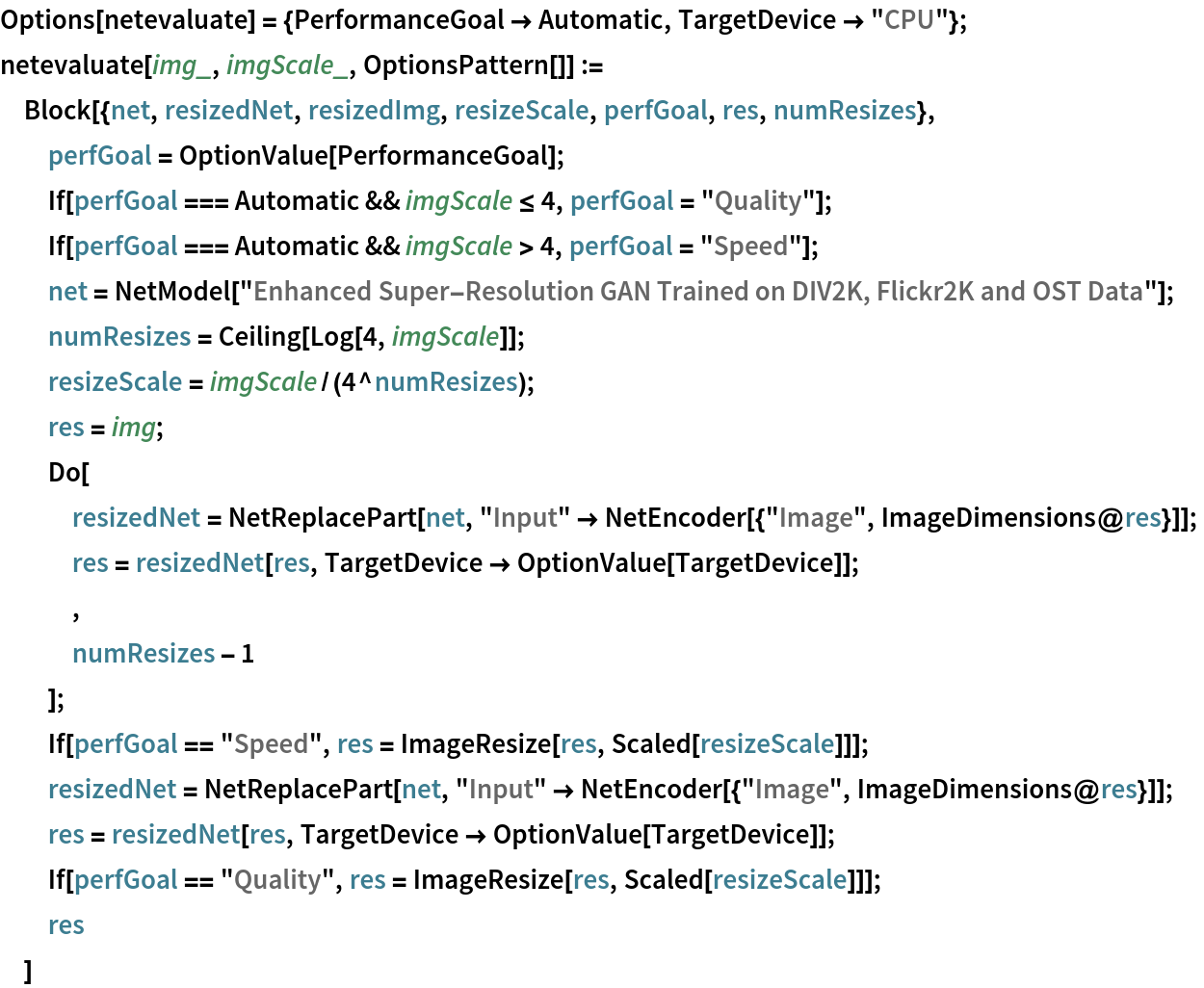### Basic usage

Get an image:

 In:=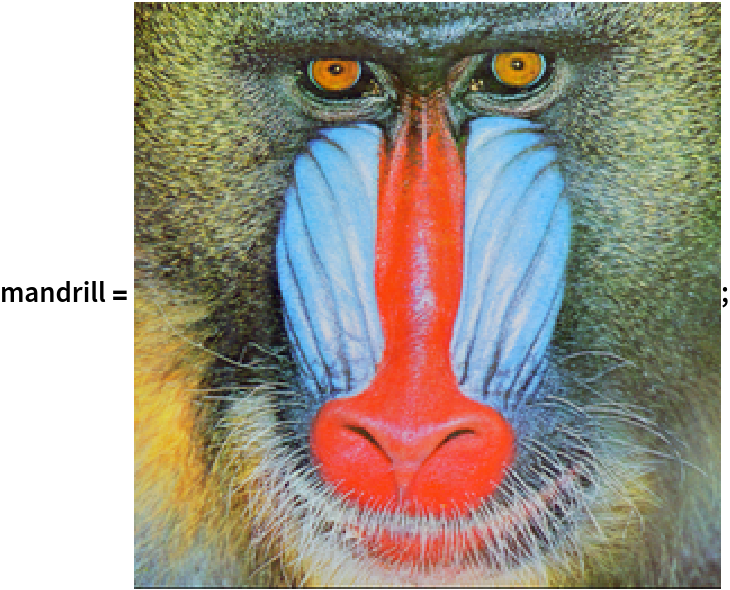Downscale the image by a factor of 3:

 In:=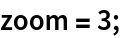In:=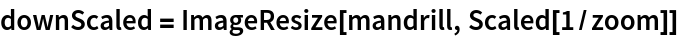Out=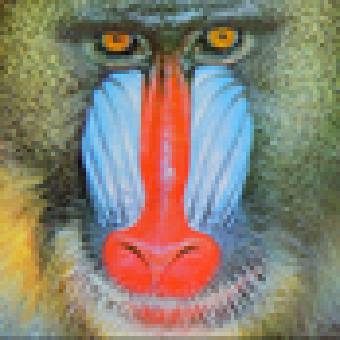Upscale the downscaled image using the net:

 In:=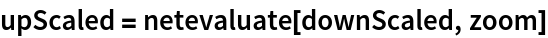Out=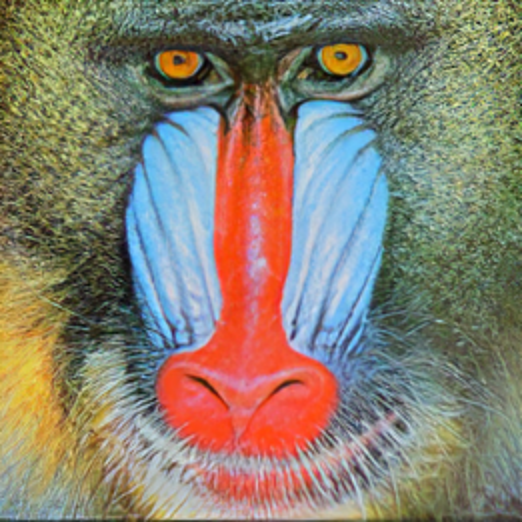Compare the details with a naively upscaled version and the original:

 In:=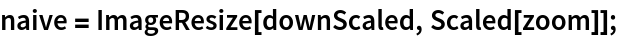In:=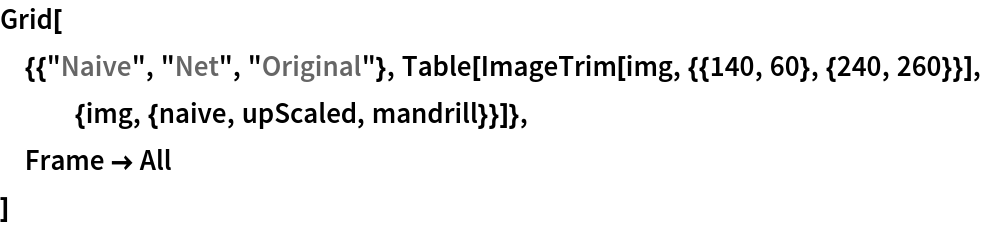Out=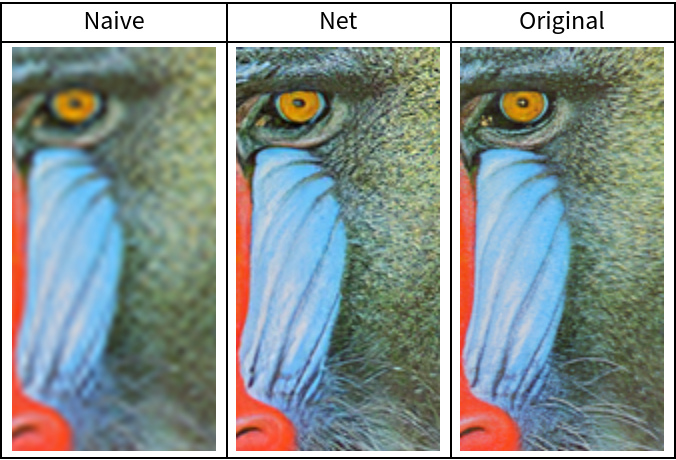Evaluate the peak signal-to-noise ratio:

 In:=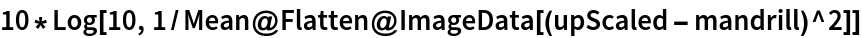Out=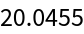When upscaling by factors of 4 or less, as in the examples in the previous section, ESRGAN is applied once. If necessary, the image is downscaled to match the required factor. It is possible to choose whether to downscale before or after ESRGAN is applied, affecting both the running time and the quality of the final result. Get an image:

 In:=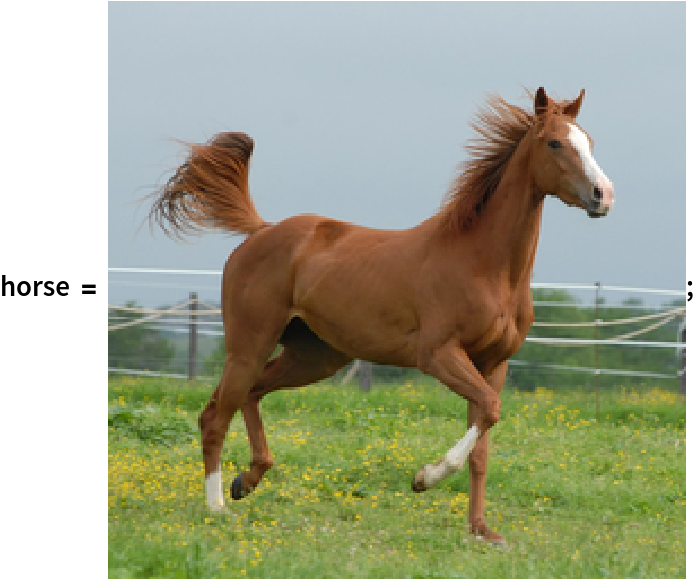Downscale the image by a factor of 3:

 In:=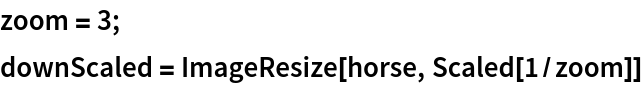Out=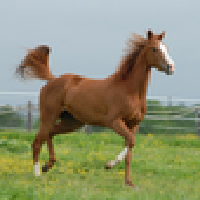When setting PerformanceGoal to "Speed", the input image is first downscaled by a factor of 3/4 and then upscaled, using ESRGAN, by a factor of 4. This will make the network operate on the smallest possible image but will throw away some details of the original, yielding a lower quality result:

 In:=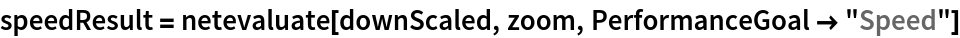Out=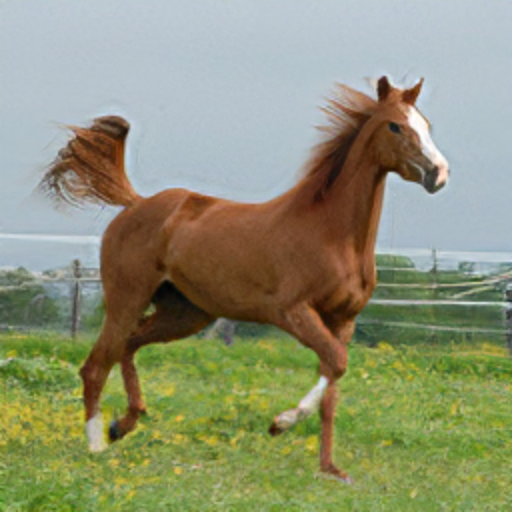When setting PerformanceGoal to "Quality", the input image is first upscaled, using ESRGAN, by a factor of 4 and then downscaled by a factor of 3/4. This is the default setting for factors of 4 or less and will make the network operate on the full-sized image, yielding a higher-quality result:

 In:=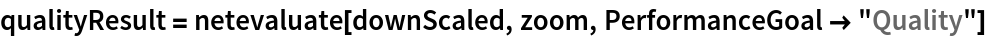Out=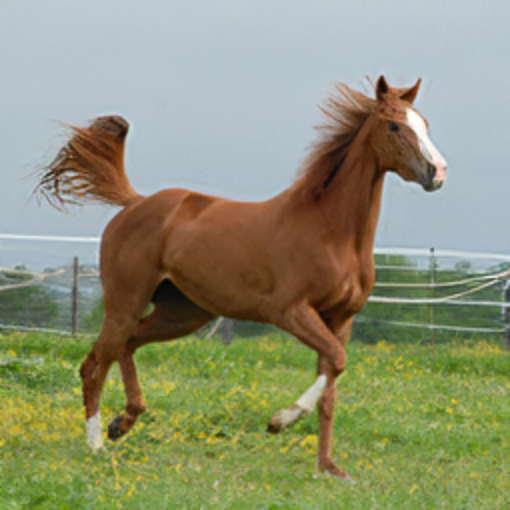Compare the details with a naively upscaled version and the original:

 In:=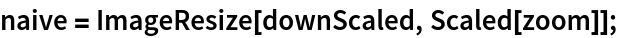In:=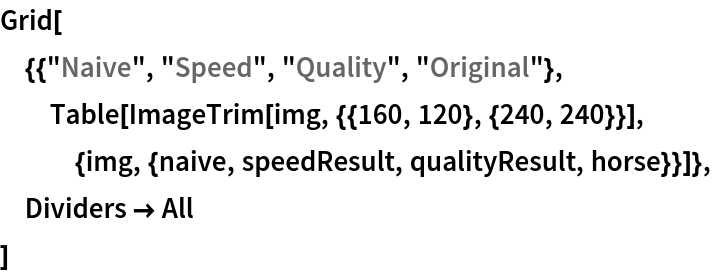Out=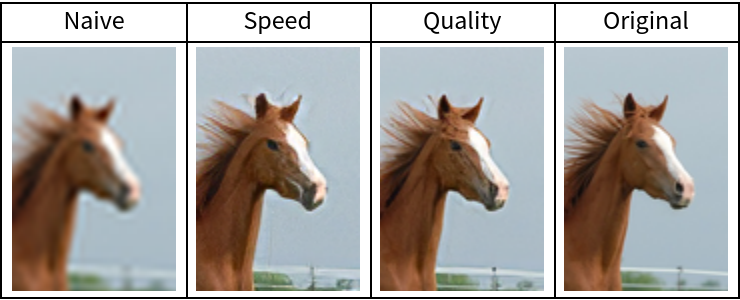When upscaling by factors of more than 4, ESRGAN must be applied multiple times. Get an image:

 In:=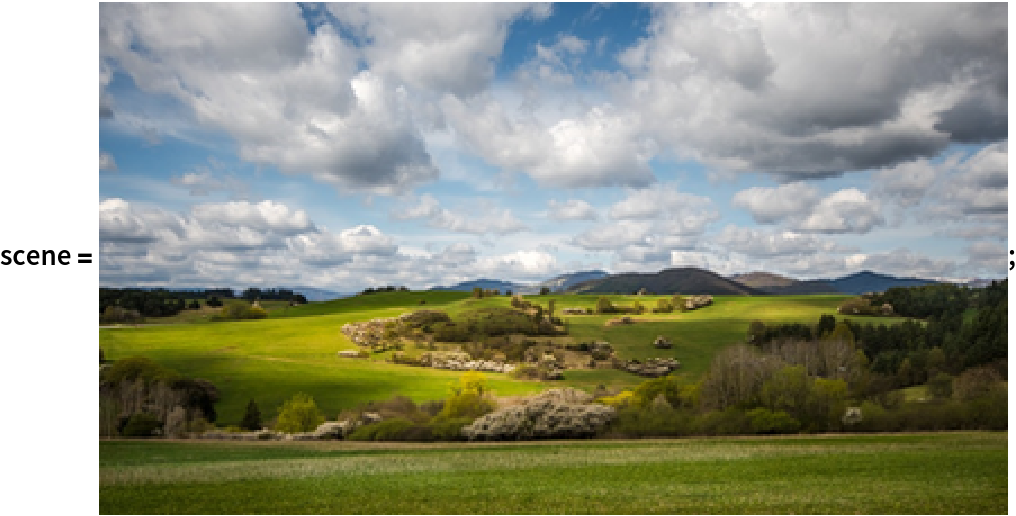Downscale the image by a factor of 6:

 In:=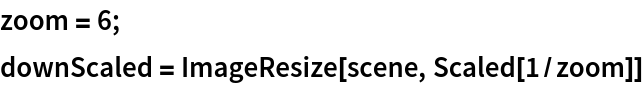Out=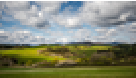When setting PerformanceGoal to "Speed", the downscaling happens before the last evaluation of ERSGAN. Hence, for a factor of 6, the input image is first upscaled by a factor of 4, then downscaled by a factor of 6/16 and finally upscaled by a factor of 4 again. This is the default setting for factors larger than 4:

 In:=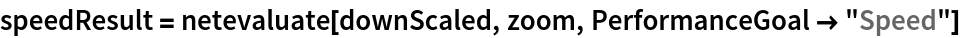Out=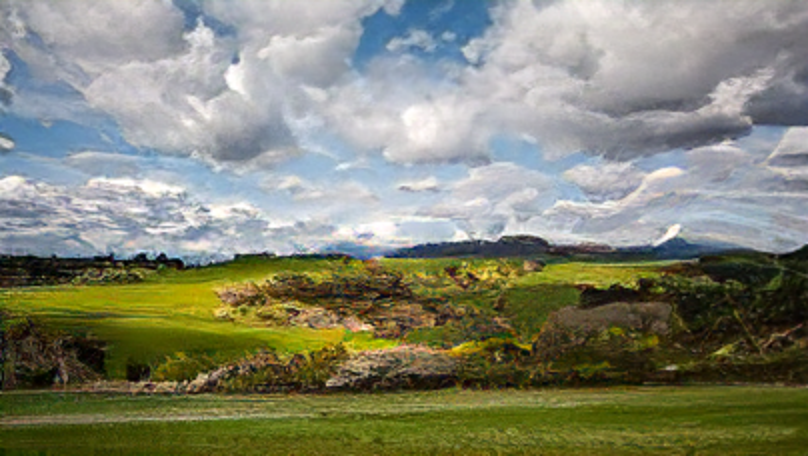When setting PerformanceGoal to "Quality", the downscaling happens after the last evaluation of ERSGAN. Hence, for a factor of 6, the input image is first upscaled by a factor of 4 twice and then downscaled by a factor of 6/16 (if available, set TargetDevice -> "GPU" for faster evaluation time):

 In:=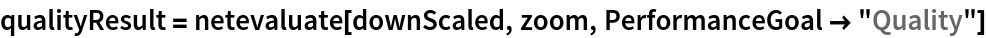Out=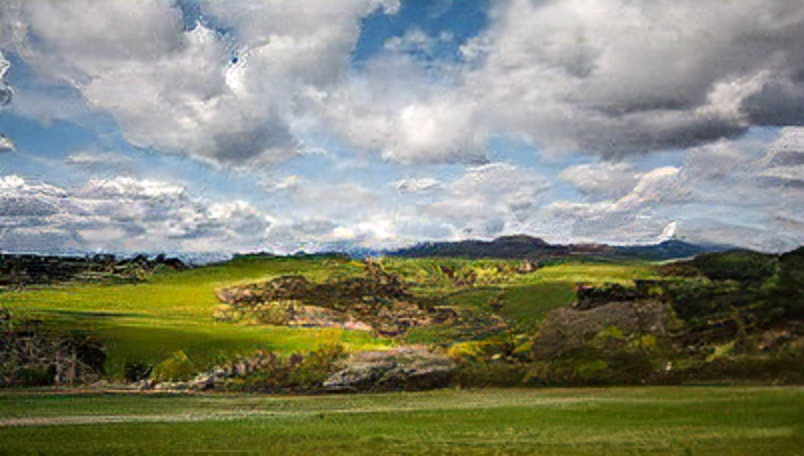Compare the details with a naively upscaled version and the original:

 In:=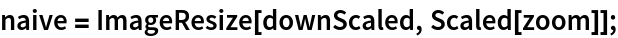In:=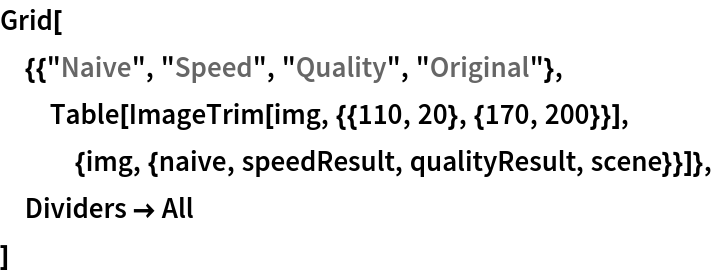Out=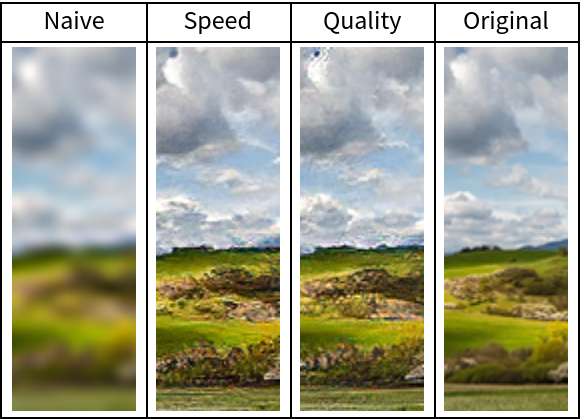### Net information

Inspect the number of parameters of all arrays in the net:

 In:=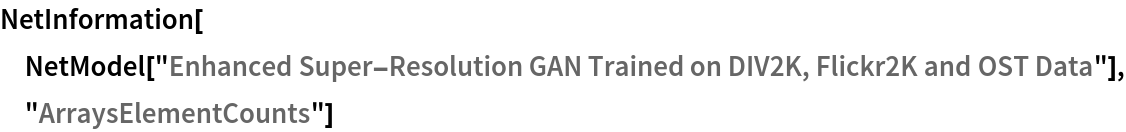Out=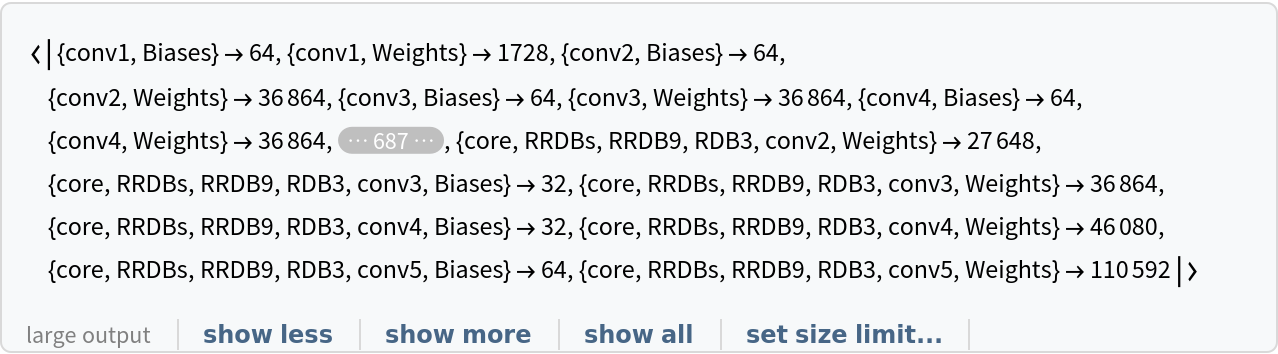Obtain the total number of parameters:

 In:=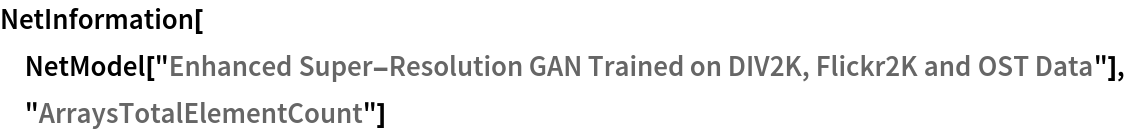Out=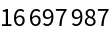Obtain the layer type counts:

 In:=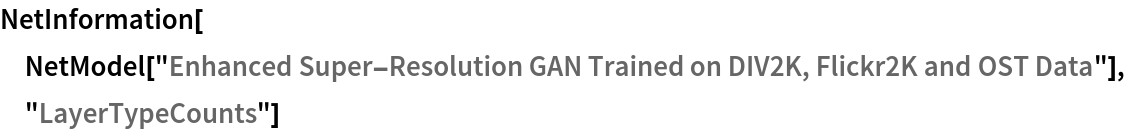Out=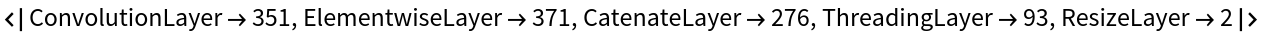Display the summary graphic:

 In:=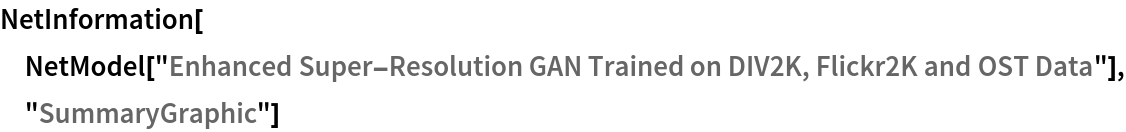Out=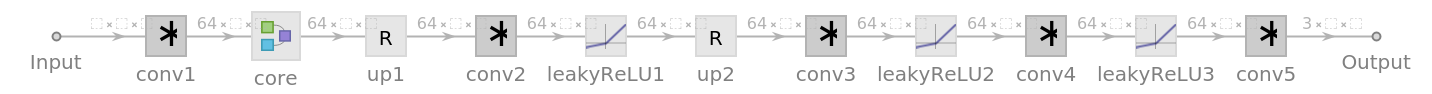### Export to MXNet

Export the net into a format that can be opened in MXNet:

 In:=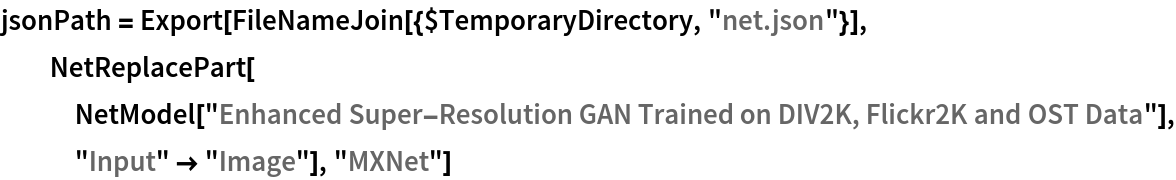Out=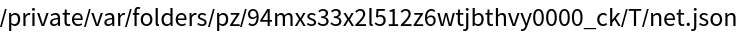Export also creates a net.params file containing parameters:

 In:=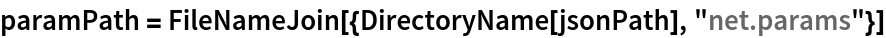Out=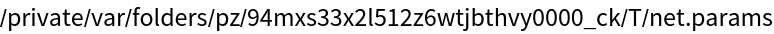Get the size of the parameter file:

 In:=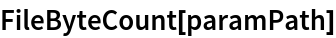Out=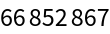The size is similar to the byte count of the resource object:

 In:=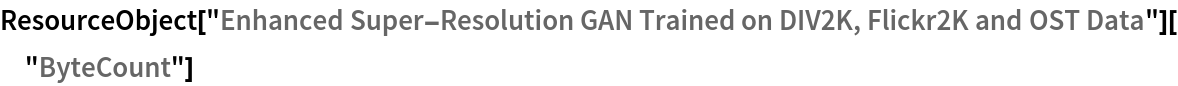Out=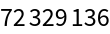## Requirements

Wolfram Language 12.0 (April 2019) or above